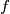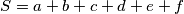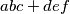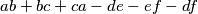### IMO Shortlist 2005 problem N3

Kvaliteta:
Avg: 3.0
Težina:
Avg: 7.0
Let$a$,$b$,$c$,$d$,$e$,$f$ be positive integers and let$S = a+b+c+d+e+f$.
Suppose that the number$S$ divides$abc+def$ and$ab+bc+ca-de-ef-df$. Prove that$S$ is composite.
Source: Međunarodna matematička olimpijada, shortlist 2005